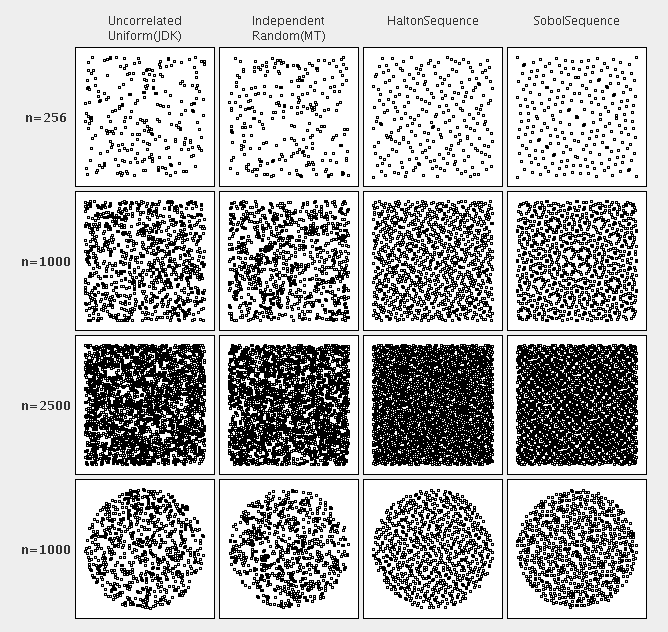## 2 Data Generation

### 2.1 Overview

Utilities in package o.a.c.m.legacy.random often uses an underlying "source of randomness": A pseudo-random number generator (PRNG) that produces sequences of numbers that are uniformly distributed within their range. Commons Math depends on Commons RNG for the PRNG implementations.

### 2.2 Correlated random vectors

Some algorithms require random vectors instead of random scalars. When the components of these vectors are uncorrelated, they may be generated simply one at a time and packed together in the vector.

When the components are correlated however, generating them is more difficult. The CorrelatedVectorFactory class provides this service. In this case, a complete covariance matrix must be provided (instead of a simple standard deviations vector) gathering both the variance and the correlation information of the probability law.

The main use for correlated random vector generation is for Monte-Carlo simulation of physical problems with several variables, for example to generate error vectors to be added to a nominal vector. A particularly common case is when the generated vector should be drawn from a Multivariate Normal Distribution.

Generating random vectors from a bivariate normal distribution:

import java.util.function.Supplier;
import org.apache.commons.rng.UniformRandomProvider;
import org.apache.commons.rng.RandomSource;

// Import common PRNG interface and factory class that instantiates the PRNG.
// Create (and possibly seed) a PRNG.
long seed = 17399225432L; // Fixed seed means same results every time
UniformRandomProvider rng = RandomSource.create(RandomSource.MT, seed);

// Create a a factory of correlated vectors.
CorrelatedVectorFactory factory = new CorrelatedVectorFactory(mean, covariance, 1e-12);
Supplier<double[]> generator = factory.gaussian(rng);

// Use the generator to generate correlated vectors.
double[] randomVector = generator.get();
... 
The mean argument is a double[] array holding the means of the random vector components. In the bivariate case, it must have length 2. The covariance argument is a RealMatrix, which has to be 2 x 2. The main diagonal elements are the variances of the vector components and the off-diagonal elements are the covariances. For example, if the means are 1 and 2 respectively, and the desired standard deviations are 3 and 4, respectively, then we need to use
double[] mean = {1, 2};
double[][] cov = {{9, c}, {c, 16}};
RealMatrix covariance = MatrixUtils.createRealMatrix(cov);

where "c" is the desired covariance. If you are starting with a desired correlation, you need to translate this to a covariance by multiplying it by the product of the standard deviations. For example, if you want to generate data that will give Pearson's R of 0.5, you would use c = 3 * 4 * 0.5 = 6.

### 2.3 Low discrepancy sequences

There exist several quasi-random sequences with the property that for all values of N, the subsequence x1, ..., xN has low discrepancy, which results in equi-distributed samples. While their quasi-randomness makes them unsuitable for most applications (i.e. the sequence of values is completely deterministic), their unique properties give them an important advantage for quasi-Monte Carlo simulations.
Currently, the following low-discrepancy sequences are supported:

// Create a Sobol sequence generator for 2-dimensional vectors
RandomVectorGenerator generator = new SobolSequence(2);

// Use the generator to generate vectors
double[] randomVector = generator.nextVector();
... 
The figure below illustrates the unique properties of low-discrepancy sequences when generating N samples in the interval [0, 1]. Roughly speaking, such sequences "fill" the respective space more evenly which leads to faster convergence in quasi-Monte Carlo simulations.